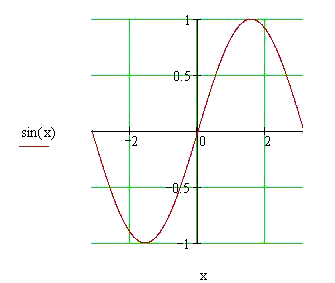Calculus on Demand at Dartmouth College Lecture 26 | Index | Lecture 28 Lecture 27Resources

Math 3 Course Syllabus
Practice Exams

Contents

In this lecture we define the inverse trigonometric functions and develop formulas for their derivatives. This will round out and conclude our discussion of the elementary functions that we began in lecture 1.

Quick Question

Does sin(x) have an inverse function on the interval [−π,π]?Textbook

Inverse Trigonometric Functions